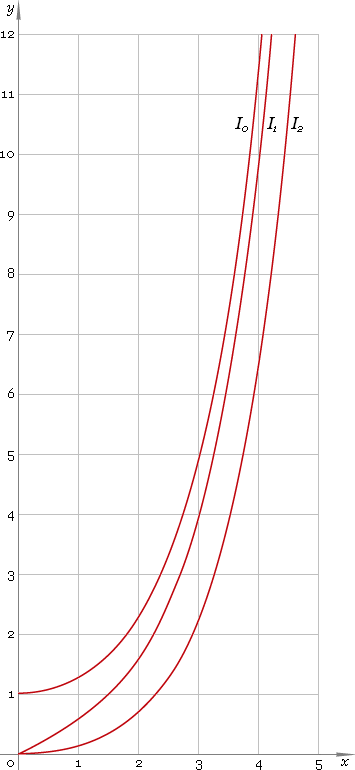The Art of Mathematics

# I — modified Bessel function of the first kind

## 1. Definition

By definition modified Bessel function is solution of the modified Bessel equation

z2 w′′ + z w′ − (z2 + ν2) w = 0

As second order equation it has two solutions, first of which has no singularity at 0 and is called modified Bessel function of the first kind — Iν. Parameter ν is called order of the function.

Second solution has singularity at 0 and is called modified Bessel function of the second kind — Kν.

## 2. Graph

Modified Bessel functions of the first kind defined everywhere on the real axis, so their domain is (−∞, +∞). Graphs of the first three representatives of the first kind modified Bessel function family depicted below — fig. 1.Fig. 1. Graphs of the modified Bessel functions of the first kind y = I0(x), y = I1(x) and y = I2(x).

## 3. Identities

Connection to the ordinary Bessel function of the first kind:

Iν(z) = (−i)νJν(iz)

Connection to the modified Bessel function of the second kind:

I−ν(z) = Iν(z) + 2 /π sin(πν) Kν(z)

Next order recurrence:

Iν+1(z) = −2ν /z Iν(z) + Iν−1(z)

Negative argument:

Iν(−z) = eiπν Iν(z) = [cos(πν) + i sin(πν)] Iν(z)

For the case of integer order ν=n the negative argument identity can be simplified down to:

In(−z) = (−1)n In(z)

and for the case of half-integer order ν=n+1/2 the identity can be simplified down to:

In+1/2(−z) = i (−1)n In+1/2(z)

Reflection — negative order:

I−ν(z) = Iν(z) + 2 /π sin(πν) Kν(z)

For the case of integer order ν=n reflection identity can be simplified down to:

I−n(z) = In(z)

and for case of half-integer order ν=n+1/2 the identity can be simplified down to:

I−n−1/2(z) = In+1/2(z) + (−1)n 2 /π Kn+1/2(z)

## 4. Derivative

Modified Bessel function of the first kind derivative:

I′ν(z) = Iν−1(z) − ν Iν(z) /z I′ν(z) = ν Iν(z) /z + Iν+1(z) I′ν(z) = [Iν−1(z) + Iν+1(z)] /2

In particular:

I′0(z) = I1(z)

## 5. Interface

Modified Bessel function call looks like:

``I(ν, z);``

where ν is the function real order, and z — function complex argument.

## 6. How to use

To calculate modified Bessel function of the first kind of the 0 order of the number:

``I(0, −1.5+i);``

To get modified Bessel function of the first kind of the 1.2 order of the current result:

``I(1.2, rslt);``

To get modified Bessel function of the first kind of the 2.5 order of the number z in calculator memory:

``I(2.5, mem[z]);``

## 6. Support

Modified Bessel function of the first kind of real order and complex argument is supported in professional version of the Librow calculator.